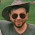## Feb 6, 2011

Thanks to Orion (Lynn Spitz), the Celestial Architect Spreadsheet now has new and more scientific temperature calculations.  There's also a new sheet that helps you calculate the planet's albedo.  I really appreciate the assistance.

Get the Open Office version here

Get the Microsoft Excel version here

1.Thank you for the update to the Celestial Architect Spreadsheet, as it has been very helpful. Do you know if it would be possible in a future update to calculate the mass of the planet given the ratio of the core to the total volume and the dominant elements that compose it? Or something along the same lines for the content of the atmosphere?

2.You are welcome.

I'd love to have something that deals with atmosphere to some degree, but that involved a much higher level of complexity. While it sounds simpler the mass thing you describe may also be beyond my abilities.

Though now that you mention it, the planet calculations probably aren't set up as conveniently as they should be. People are probably more interested in gravity and radius as inputs. Mass should be one of the derived stats -- if it didn't make the math so much trickier.

3.Given radius at surface and gravity at surface, it should be easy to calculate mass? a = G M / r squared etc.
Then generating other details can continue as normal.

Incidentally there is a practical virtual world implementation at pioneer, with terrain types, oceans, orbits in solar systems etc. It is a procedurally generated space sim that is opensource similar to infinity at early alpha release. It could be further detailed out

http://pioneerspacesim.net/, SSC forums, and #pioneer on freenode.

4.Calculating the mass of a planet only requires that you know its radius and its surface gravity, because surface gravity is proportional to the radius times the bulk density. So, G/R will give you the density. Once you have the density, then the mass is simply the density times the volume, i.e., D * R cubed. The math is simplest if you express all quantities relative to the values for Earth, then you don't have to futz around wit constants like pi or convert between units.

Metalraptor, what did you mean by "the content of the atmosphere"? The mass of the atmosphere? The surface pressure? The scale height? The chemical composition? Most people are interested only in the last three quantities, as they determine whether or not you can breath it, and how high up it is breathable. The total atmospheric mass is easily calculated from the surface pressure and the gravity, but it's not of much practical interest to most world-builders.

5.Orion: yeah, you are right. It isn't actually very tricky to work from Gravity and radius. Thanks for pointing that out.
Those will be the directly user-adjustable variables in the next release.

I also got an oversimplified but (i think) roughly valid calculations for binary systems in the works